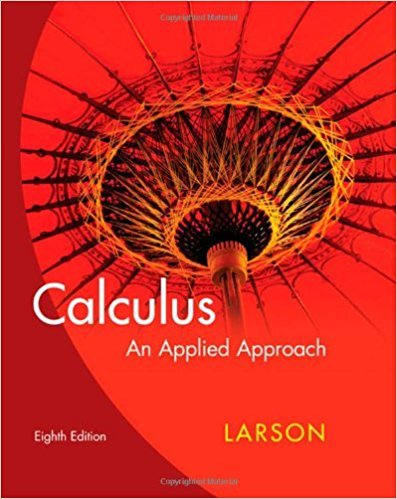×
×

# Solutions for Chapter 0.5: Fractions and Rationalization## Full solutions for Calculus: An Applied Approach | 8th Edition

ISBN: 9780618958252Solutions for Chapter 0.5: Fractions and Rationalization

Solutions for Chapter 0.5
4 5 0 311 Reviews
26
2
##### ISBN: 9780618958252

Since 48 problems in chapter 0.5: Fractions and Rationalization have been answered, more than 22539 students have viewed full step-by-step solutions from this chapter. Calculus: An Applied Approach was written by and is associated to the ISBN: 9780618958252. Chapter 0.5: Fractions and Rationalization includes 48 full step-by-step solutions. This textbook survival guide was created for the textbook: Calculus: An Applied Approach , edition: 8. This expansive textbook survival guide covers the following chapters and their solutions.

Key Calculus Terms and definitions covered in this textbook
• Arcsecant function

See Inverse secant function.

• Binomial coefficients

The numbers in Pascal’s triangle: nCr = anrb = n!r!1n - r2!

• Boundary

The set of points on the “edge” of a region

• Continuous function

A function that is continuous on its entire domain

• Cubic

A degree 3 polynomial function

• Divisor of a polynomial

See Division algorithm for polynomials.

• Elements of a matrix

See Matrix element.

• Endpoint of an interval

A real number that represents one “end” of an interval.

• Equation

A statement of equality between two expressions.

• equation of a hyperbola

(x - h)2 a2 - (y - k)2 b2 = 1 or (y - k)2 a2 - (x - h)2 b2 = 1

• Equilibrium point

A point where the supply curve and demand curve intersect. The corresponding price is the equilibrium price.

• Identity

An equation that is always true throughout its domain.

• nth root

See Principal nth root

• One-to-one rule of logarithms

x = y if and only if logb x = logb y.

• Parametric curve

The graph of parametric equations.

• Polar axis

See Polar coordinate system.

The formula x = -b 2b2 - 4ac2a used to solve ax 2 + bx + c = 0.

The distance from a point on a circle (or a sphere) to the center of the circle (or the sphere).

• Remainder polynomial

See Division algorithm for polynomials.

• Stretch of factor c

A transformation of a graph obtained by multiplying all the x-coordinates (horizontal stretch) by the constant 1/c, or all of the y-coordinates (vertical stretch) of the points by a constant c, c, > 1.

×